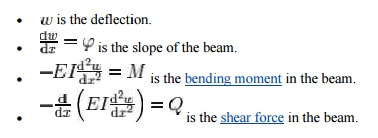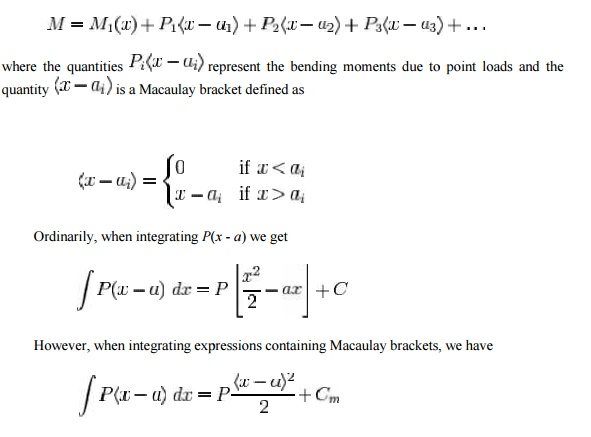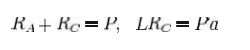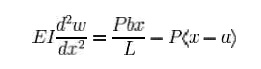Home | | Mechanics of Solids | Evaluation of beam deflection and slope

# Evaluation of beam deflection and slopeStatic beam equation Bending of an Euler-Bernoulli beam. Each cross-section of the beam is at 90 degrees to the neutral axis.

Evaluation of beam deflection and slope

Beam deflection

Static beam equationBending of an Euler-Bernoulli beam. Each cross-section of the beam is at 90 degrees to the neutral axis.

The Euler-Bernoulli equation describes the relationship between the bea m's deflection and the applied load.The curve w(x) describes the d eflection w of the beam at some position x (recall that the beam is modeled as a one-dimensionall object). q is a distributed load, in other words a force per unit length (analogous to pressure being a force per area); it may be a funct ion of x, w, or other variables.

Note that E is the elastic modu lus and that I is the second moment of area . I must be calculated with respect to the centroidal axis perpendicular to the applied loading. F or an Euler-Bernoulli beam not under any axial loadi ng this axis is called the neutral axis.

Often, w = w(x), q = q(x), and EI is a constant, so that:This equation, describing the d eflection of a uniform, static beam, is used widely in engineering practice. Tabulated expression s for the deflection wfor common beam configurations can be found in engineering handbo oks. For more complicated situations the deflection can be determined by solving the E uler-Bernoulli equation using techniques such as the "slope deflection method", "moment distribution method", "moment area method, "conjugate beam method", "the principle of virtual work", "direct integration", "Ca stigliano's method", "Macaulay's method" or the "di rect stiffness method".

Successive derivatives of w ha ve important meanings:The stresses in a beam can be calculated from the above expressions after t he deflection due to a given load has been determined .

A number of different sign co nventions can be found in the literature on the bending of beams and care should be taken to maintain consistency.In this article, the sign convention has been chosen so the coordinate system is right handed. Forces acting in the posi tive x and z directions are assumed positive. The sign of the bending moment is chosen so that a p ositive value leads to a tensile stress at the bottom cords. The sign of the shear force has bee n chosen such that it matches the sign of the bending moment.

Double integration metho d

The double integration method  is a powerful tool in solving deflection and slope of a beam at

any point because we will be a ble to get the equation of the elastic curve.

Thus, EI / M = 1 / y''

.

Macaulay Method

The starting point for Maucaulay's method is the relation between bending moment and curvature from Eu ler-Bernoulli beam theoryThis equation is simpler tha n the fourth-order beam equation and can b e integrated twice to find w if the value of M as a function of x is known. For general loadings, M can be expressed in the formwith the difference between th e two expressions being contained in the constant Cm. Using these integration rules makes the c alculation of the deflection of Euler-Bernoulli beams simple in situations where there are m ultiple point loads and point moments. Th e Macaulay method predates more sophisticated concepts such as Dirac delta functions a nd step functions but achieves the same outcomes for beam problems.

Example: Simply supported beam with point loadSimply supported beam with a single eccentric concentrated load.

An illustration of the Macaulay method considers a simply supported beam with a single eccentric concentrated load as shown in the adjacent figure. The first step is to find M. The reactions at the supports A and C are determined from the balance of forces and moments asTherefore RA = Pb / L and the b ending moment at a point D between A and B (0 < x < a) is given by

M = RAx = Pbx / L

Using the moment-curvature relation and the Euler-Bernoulli express ion for the bending moment, we haveFor a point D in the region BC (a < x < L), the bending moment is

M = RAx - P(x - a) = Pbx / L - P(x - a)

In Macaulay's approach we us e the Macaulay bracket form of the above ex pression to represent the fact that a point load has been applied at location B, i.e.,Comparing equations (iii) & (vii) and (iv) & (viii) we notice that due to continuity at point B, C1 = D1 and C2 = D2. The a bove observation implies that for the two regions considered, though the equation for bending moment and hence for the curvature are d ifferent, the constants of integration got during successive integration of the equation for curvatur e for the two regions are the same.

The above argument holds true for any number/type of discontinuities in the equations for curvature, provided that in each case the equation retains the term for the subsequent region in the form (x-a)n,(x-b)n,(x-c)n   etc. It should be remembered that for any x, giving the  quantities  within  the  brac kets,  as in  the  above  case,  -ve  should  b e  neglected,  and  the calculations should be made considering only the quantities which give +ve sign for the terms within the brackets.

Reverting back to the problem, we haveIt is obvious that the first term only is to be considered for x < a and both t he terms for x > a and the solution isNote that the constants are placed immediately after the first term to indicate that they go with the first term when x < a and with both the terms when x > a. The Macaulay brackets help as a reminder that the quantity on th e right is zero when considering points with x < a.

Study Material, Lecturing Notes, Assignment, Reference, Wiki description explanation, brief detail
Civil : Mechanics Of Solids : Deflection Of Beams : Evaluation of beam deflection and slope |